## Frank ICSE Solutions for Class 9 Physics – Light: Spherical Mirrors

PAGE NO: 258
Solution 1:
A spherical mirror is a part of a hollow glass sphere silvered on one side.

Solution 2:Solution 3:
Focal length = 1/2 of radius of curvature
= 1/2 x 30 = 15cm.

Solution 4:
Focal point is the principal focus of the mirror where a parallel beam of light meets(or appear to meet) after reflection from the mirror.

Solution 5:Solution 6:

• Pole is the centre of the reflecting surface, in this case spherical mirror.
• Centre of curvature is the centre of the imaginary sphere to which the mirror belongs
• Aperture is the distance between the extreme points on the periphery of the mirror.
• Principal axis is the straight line passing through the pole and the centre of curvature.
• The principle focus of a spherical mirror may be defined as a point on its principle axis where a beam of light parallel to the principle axis converges to or appears to diverge from after reflection from the spherical mirror.

Solution 7:
Convex mirror has a wider field of view.

Solution 8:
Concave mirrors are used in reflecting microscope, in shaving and make up glasses and in ophthalmoscope.

Solution 9:
Convex mirrors are used as a rear view mirror in automobiles as it provides a wider view of following traffic.

Solution 10:
Convex mirror is used in vehicles to see the traffic following it.

Solution 11:
The relationship between the focal length, f and radius of curvature, r is
f = 1/2 x r.

Solution 12:Solution 13: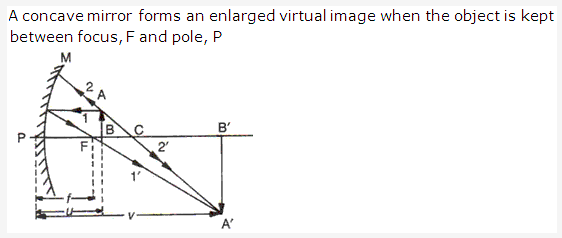Solution 14:
Concave mirror can produce real and diminished image of the object.

Solution 15:
The focal length of plane mirror is infinity.

Solution 16:
The object should be placed between F and P to obtain its magnified and erect image.

Solution 17: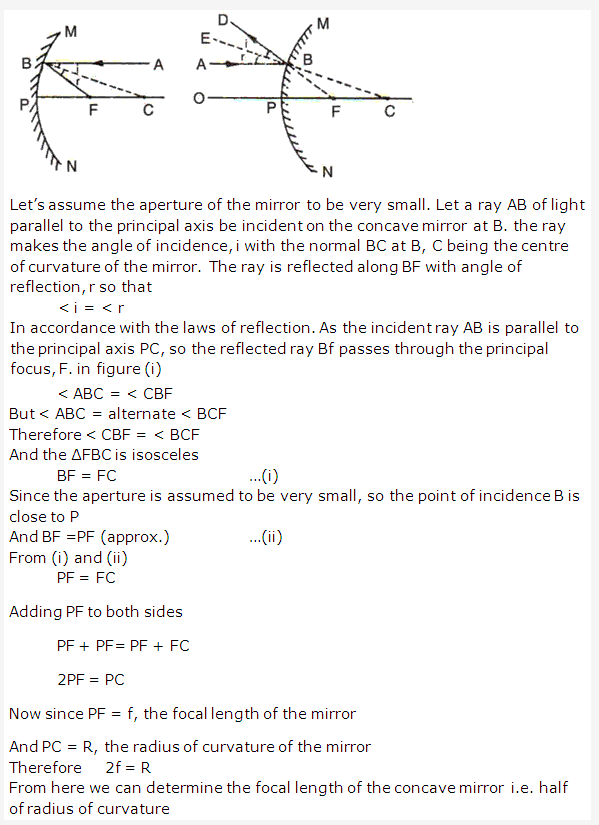Solution 18:
Linear magnification is defined as the ratio of the height of the image to the height of the object. It is taken to be positive for an image to be virtual and erect and negative when image is real and inverted.
Magnification = height of image / height of object.

Solution 19:
SI unit of focal length is meter.

Solution 20:
The top mirror is convex mirror, the middle mirror is concave mirror and bottom mirror is a plane mirror.

Solution 21:
The mirror having +15 cm as its focal length is a convex mirror because focal length is taken positive only in case of convex mirror.

Solution 22:
The mirror having -20 cm as its focal length is a concave mirror because focal length is taken negative only in case of concave mirror.

Solution 23:
When we look into a plane mirror, the image of our face is virtual because the image cannot be obtained on a screen.

Solution 24:
When an object is brought towards the concave mirror, the position of the image moves away from the mirror and the size increases and it remains inverted but at object position between F and P, the image is virtual, magnified and erect.

Solution 25:PAGE NO : 259
Solution 26: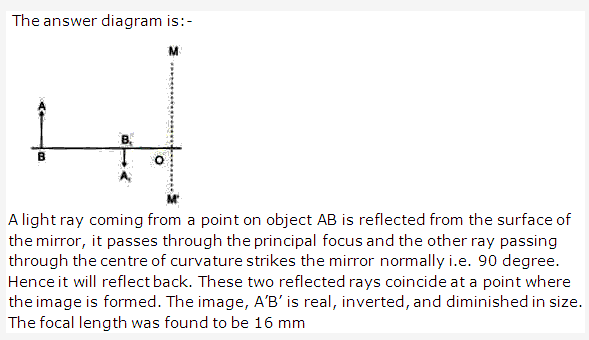Solution 27: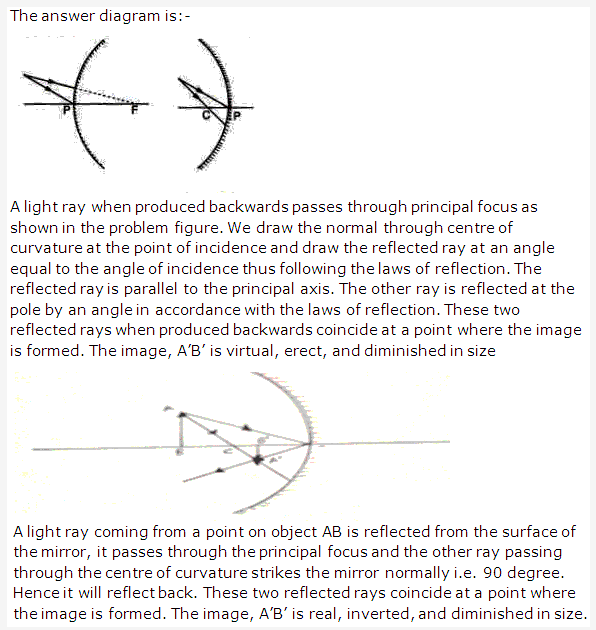Solution 28: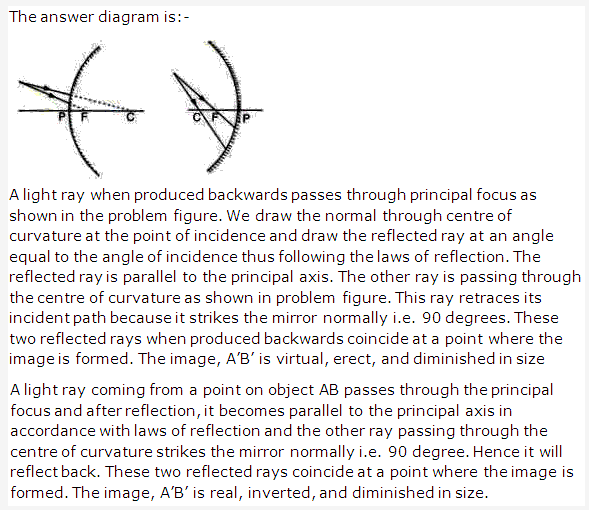Solution 29:Solution 30:## Frank ICSE Solutions for Class 9 Physics – Light

PAGE NO: 261
Solution 1:
Convex mirror has a wider field of view.

Solution 2:
Convex mirror always produces an erect image of the object.

Solution 3:
Convex mirror is used in vehicles to see the traffic on rear side.

Solution 4:
We will use convex mirror to see an enlarged image of our face.

Solution 5:
Image of object placed at a long distance in front of a convex mirror is formed at principal focus. Radius of curvature of convex mirror is 20 cm.
Focal length of convex mirror = radius of curvature/2.
Focal length of convex mirror = 20/2 = 10 cm.
So image will form at principal focus 10 cm away from pole.

Solution 6:
Concave mirror can produce real and diminished image of the object.

Solution 7:
The distance of the principal focus from the pole of the mirror is called the focal length of the mirror.

Solution 8:
The mirror having +20 cm as its focal length is a convex mirror because focal length is taken positive only in case of convex mirror.

Solution 9:
The focal length of plane mirror is infinity.

Solution 10:
The mirror having -15 cm as its focal length is a concave mirror because focal length is taken negative only in case of concave mirror.

Solution 11:
Principal axis is the straight line passing through the pole and the centre of curvature.

Solution 12:
Linear magnification is defined as the ratio of the height of the image to the height of the object. It is taken to be positive for an image to be virtual and erect and negative when image is real and inverted.
Magnification = height of image / height of object.

Solution 13:
Pole is the centre of the reflecting surface, in this case spherical mirror.

Solution 14:
Centre of curvature is the centre of the imaginary sphere to which the mirror belongs.

Solution 15:
Three characteristics of light are:-

• Light waves can travel through vacuum.
• Light waves are transverse waves.
• The velocity of light in vacuum is 3 x 108 m/s.

Solution 16:
Three distinctions between light and sound waves are

• Light waves can travel through vacuum while sound waves cannot.
• Light waves are transverse waves while sound waves are longitudinal waves.
• The velocity of light in air is 3 x 108 m/s while the speed of light in air is just about 330 m/s.

Solution 17:

• When position of object is at infinity, concave mirror forms a point and Real image at Focus point.
• When position of object is beyond C, concave mirror forms a Diminished, Real and inverted image between F and C.
• When position of object is at C, concave mirror forms a Magnified, Real and inverted image at C.

Solution 18:
Image formed by a convex mirror is always Diminished, Virtual and Erect.

Solution 19:
Concave mirrors are used in reflecting microscope, in shaving and make up glasses and in ophthalmoscope.

Solution 20:

• The distance from the pole in the direction of incident ray is taken positive.
• The distance from the pole in the direction opposite to the incident ray is taken negative.

Solution 21:
Mirror formula is the relation between the focal length f of the mirror, the distance u of the object from the pole of the mirror, and the distance v of the image from the pole.
Mirror formula is
1/v +1/u = 1/f.
Linear magnification is defined as the ratio of the height of the image to the height of the object. It is taken to be positive for an image to be virtual and erect and negative when image is real and inverted.
Magnification = height of image / height of object.

Solution 22:
Mirror formula is the relation between the focal length f of the mirror, the distance u of the object from the pole of the mirror, and the distance v of the image from the pole.
Mirror formula is
1/v +1/u = 1/f.
Size of body = 1.5 m.
Magnification of body = 1.5.
Magnification = height of image / height of object.
Height of image = magnification x height of object.
Height of image = 1.5 x 1.5= 2.25 m.

Solution 23:
Linear magnification is defined as the ratio of the height of the image to the height of the object. It is taken to be positive for an image to be virtual and erect and negative when image is real and inverted.
Magnification produced by concave mirror is:
Magnification = height of image / height of object.
It is a pure ratio and does not have any units.

Solution 24:Solution 25:
A smooth and polished surface causes regular reflection while a rough and unpolished surface causes irregular reflection.

Solution 26:
When rays of light fall on a surface, they are turned back into the same medium in accordance with some definite laws. This process is known as reflection.
Reflection obeys following two laws

• The incident ray, the reflected ray, and the normal at the point of incidence, all lie in the same plane.
• The angle of incidence and the angle of reflection are always equal.

Solution 27:
You can distinguish between plane mirror, a concave mirror, and a convex mirror without touching them. When you look into these mirrors by bringing your face close to each mirror, they will produce an image of your face of different types.

• A plane mirror will produce an image of the same size as your face.
• A concave mirror will produce a magnified image of your face.
• A convex mirror will produce Diminished image of your face.

Solution 28:
You can distinguish between a concave mirror and a convex mirror without touching them. When you look into these mirrors by bringing your face close to each mirror, they will produce an image of your face of different types.

• A concave mirror will produce a magnified image of your face.
• A convex mirror will produce Diminished image of your face.

PAGE NO : 262
Solution 29: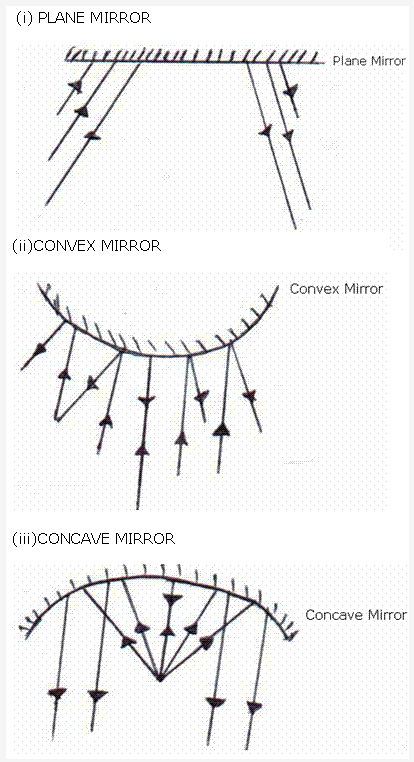Solution 30:
Uses of concave mirror:

• Concave mirrors are used in reflecting microscope
• Concave mirrors are used in shaving and make up glasses.
Uses of convex mirror: Convex mirrors are used as a rear view mirror in automobiles as it provides a wider view of following traffic.

Solution 31:
We can see the reflection of our face on a polished table top because a regular reflection occurs in case of a polished surface while on a unpolished table top irregular reflection occurs which make image of our face unclear.

Solution 32:

• The angle of incidence is the angle made by the incident ray with the plane mirror. {FALSE}
Correct statement is the angle of incidence is the angle made by the incident ray with the normal to the surface of plane mirror.
• If a ray of light incident on a plane mirror is such that it makes an angle of 30° with the normal, then the angle of reflection is 60°.{FALSE}
Correct statement is if a ray of light incident on a plane mirror is such that it makes an angle of 30° with the normal, then the angle of reflection is 30°.
• If the incident ray makes an angle of X° with the normal, then the angle between the incident ray and reflected ray is 2X°. {TRUE}
• The image formed in a plane mirror is real, erect and same size as that of the object. {FALSE}
Correct statement is the image formed in a plane mirror is virtual, erect and same size as that of the object.

Solution 33: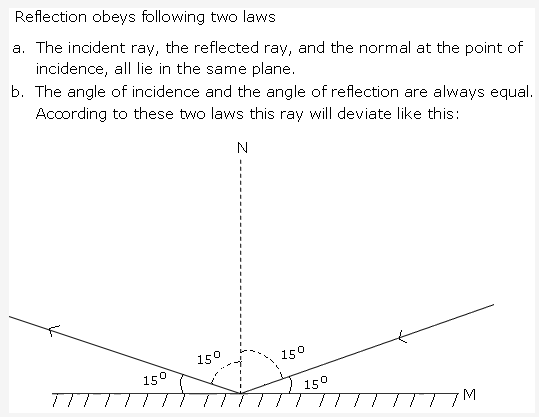Solution 34:Solution 35:
The image formed by a plane mirror is erect and virtual. It is a laterally inverted image. The image formed is of the same size as that of the object. Also, the image and the object are equidistant from the mirror.

Solution 36:Solution 37:
Given, distance of boy from the mirror = 3 m

• Distance of image from mirror = distance of boy from the mirror = 3 m
Distance between boy and his image = distance of boy from the mirror + distance of image from mirror = 3+3 = 6 m
• Now, distance of boy from the mirror = 4 m
Distance of image from mirror = 4 m
Distance between boy and his image = distance of boy from the mirror + distance of image from mirror = 4+4 = 8m.

Solution 38:
Periscope is used to see over the top of an obstacle. It is also used in submarines for observing for movement of ships. It can be used from the trenches for observing the movement on the surface of earth.

PAGE NO : 263
Solution 39:Solution 40:

• Pole is the centre of the reflecting surface, in this case spherical mirror.
• Centre of curvature is the centre of the imaginary sphere to which the mirror belongs
• Principal focus of a spherical mirror is a point on the principal axis of the mirror, where all the rays travelling parallel to the principal axis and close to it after reflection from the mirror, converge to or appear to diverge from.
• Principal axis is the straight line passing through the pole and the centre of curvature.
• Focus of a concave mirror is a point on the principal axis of the mirror, where all the rays travelling parallel to the principal axis and close to it after reflection from the mirror converge to that point.
• Normal to the surface of a mirror at any point is the straight line at right angle to the tangent drawn at that point.

Solution 41:Solution 42:Solution 43:Solution 44:Solution 45:Solution 46:PAGE NO : 264
Solution 47:Solution 48: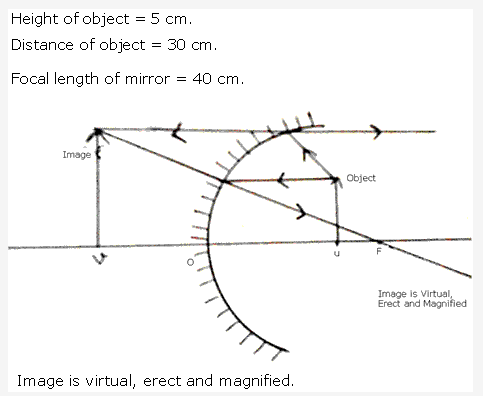Solution 49: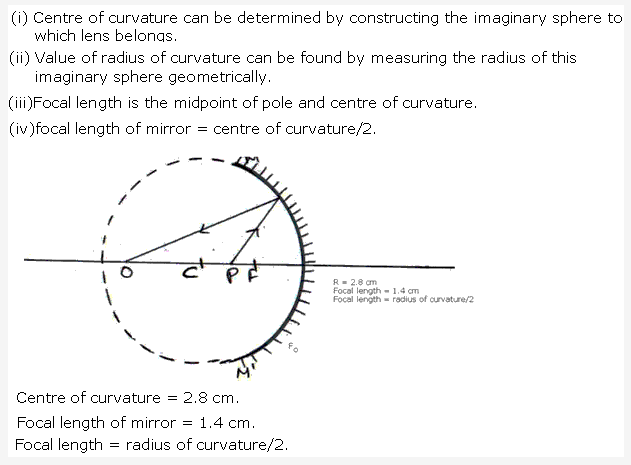Solution 50:## Frank ICSE Solutions for Class 9 Physics – Sound: Production and Propagation of Sound

PAGE NO: 278
Solution 1:
Yes, Sound is form of energy.

Solution 2:
Sound is produced by the vibrating objects.

Solution 3:
No, sound cannot travel through vacuum.

Solution 4:
Yes, sound can propagate through solids and liquids.

Solution 5:
No, sound cannot be produced in vacuum. It needs a material medium.

Solution 6:
Speed of sound depends on the density, pressure, temperature, humidity and Wind.

Solution 7:
330m/s is the value of speed in air at N.T.P

Solution 8:
In water, speed of sound is 4.5 times more than that in air. And, in iron, the speed of sound is 15 times as fast in air.

Solution 9:
The speed of sound is more in the humid air as compared to dry air. Because, the air moisture decreases the density of air and speed of sound is inversely proportional to density of air. So, sound travels faster in humid air.

Solution 10:
Speed of sound does not depend on the amplitude and wavelength.

Solution 11:
The sound produced by the moving wheels of the train travels faster on the iron track than in the air. So, firstly sound produced because of railway track is heard and then heard in the air.

Solution 12:
The waves in the air causes sound . Through waves , energy get transferred from one place to another and hence the sound.

Solution 13:
The ratio of speed of light to the speed of sound is 9.1 x 105.

Solution 14:
Speed of sound in air is independent of variation of pressure.

Solution 15:
The speed of sound in air increases with increase in temperature because with increase in temperature, the density of air gases decreases and speed of air is inversely proportional to density of air.

Solution 16:
The diver will hear the sound first because the sound travels faster in water than in air.

Solution 17:
The simple experiment that a person can do to calculate the speed of sound in air is that a person stands at a known distance (d meter) from the cliff and fires a pistol and simultaneously start the stop watch. He stops the stop-watch as soon as he hears a echo. The distance travelled by the sound during the time (t) seconds is 2d.
So, speed of sound = distance travelled / time taken = 2d/t

Solution 18:

• Moisture in Air: The speed of sound increase with increase in humidity because the moisture in air decrease the density of air and speed of air is inversely proportional to density of air. Hence , the sound travels faster in moist air.
• Pressure in air: Speed of sound is independent of the air pressure.
• The speed of sound increases with the increase in temperature of the gas.

Solution 19:
The distance of flash from the observer = speed in air x time taken for the first sound to reach 330 x 6 = 1980m

Solution 20:
Distance between boys = speed of sound x time taken to reach sound = 330 x 2.5 = 825m

## Frank ICSE Solutions for Class 9 Physics – Sound: Range of Hearing

PAGE NO: 284
Solution 1:
The frequency of sound is defined as the number of vibrations produced by the sound producing object per second.

Solution 2:
S.I unit of frequency is hertz.

Solution 3:
One hertz is defined as one vibration produced in one second by sound producing object.

Solution 4:
The audible range of frequency is 20Hz to 20KHz.

Solution 5:

• Less than 20Hz – we cannot hear sound.
• More than 20KHz – we cannot hear sound.

Solution 6:
No, the audible range of frequency does not vary from person to person and also with age of person.

Solution 7:
Sound having frequency less than 20Hz is called infrasonic sound. Sound having frequency greater than 20KHz is called ultrasonic sound.

Solution 8:
The energy carried by ultrasonic sound is very high.

Solution 9:
Applications of Ultrasonic sound

• Ultrasonic sound is used to detect the flaws in metal castings of automobile tyres.
• It is used in hospitals to detect defects in certain parts of body.

Solution 10:
Echocardiography is used to obtain the image of the heart by the used of ultrasonic waves.

Solution 11:
SONAR stands for Sound Navigation and Ranging. Sonar helps the oceanographers and ship captains to determine the depth of the ocean.

Solution 12:
Bats emit the high frequencies that range between 20 KHz to 75 KHz. The echoes from these sounds help in locating the obstacles in their path and to avoid them.

Solution 13:
The elephants and whales produce the sound of frequency less than 20Hz. (means infrasonic sounds)

Solution 14:
No, all animals does not have same range of audible frequencies.

Solution 15:
When the sound strike any object and reflect back, the hearing of reflected sound is called echo.

Solution 16:
Echolocation is defined as sending out sounds to judge the location, size and motion of objects from the returning echoes. The animals use this phenomenon to locate the obstacles in their path and avoid them so it helps them in navigation and to locate their prey.

Solution 17:
Three Important uses of echolocation:

• It helps animals to communicate with each other. e.g dolphins use the echolocation to communicate with other dolphins.
• It helps in navigation and to locate their prey.
• It is used by them to hunt for the meal.

Solution 18:
A hearing aid is the device which increases the loudness and intensity of the incoming sound.

Solution 19:
The main components of hearing aid are microphone , amplifier and earphone.

Solution 20:
A ship sends ultrasonic waves downward in the ocean. When the sound waves reaches the ocean floor, they are reflected back to the ship . The time it takes for the signal to return is used to calculate the depth of submerged ship in the ocean.

Solution 21:
High Power, High Energy and good directionality are the properties of ultrasonic sounds which makes them useful.

Solution 22:

• 10 Hz – Infrasonic sound.
• 200 Hz – audible sound.
• 2000 Hz – Audible sound.
• 45 KHz – Ultrasonic sound

## Frank ICSE Solutions for Class 9 Physics – Sound

PAGE NO: 286
Solution 1:
Yes, we can hear in the dark room.

Solution 2:
Sound is produced by the vibrating objects.

Solution 3:
Yes, sound is a form of energy.

Solution 4:
No, sound cannot travel through vacuum. It needs a material medium to travel.

Solution 5:
Yes, sound requires a material medium for transmission.

Solution 6:
The speed of sound depends on the following factors: Density of air, Temperature , Humidity in air as well the wind.

Solution 7:
There is no effect on the wavelength and amplitude on the speed of sound.

Solution 8:
Speed of sound at room temperature is 330 m/s

Solution 9:
Yes, the speed of sound is different in different medium.

Solution 10:
No, the speed of sound in the gas is independent on the change in pressure of the gas.

Solution 11: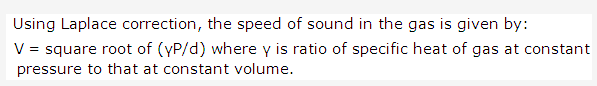Solution 12:
Yes, the speed of sound depends on the direction of wind. When wind is blowing in the direction of propagation of sound , the speed of sound increases. But, when wind blows in the direction opposite to that of sound , the speed of sound decreases.

Solution 13:
Ultrasonic are sounds having frequency higher that 20 KHz and not audible to the human being.

Solution 14:
SONAR stands for the “SOund Navigation And Ranging”.

Solution 15:
Ultrasonic sound has higher frequency than infrasonic sound.

Solution 16:
No, we cannot hear the sound produced due to the vibration of second’s pendulum.

Solution 17:
No, SONAR does not make use of infrasonic waves. Instead, it make use of ultrasonic waves.

Solution 18:
Yes, ultrasound in the medium travels faster than the audible sound.

Solution 19:
No, it is not possible to hear the friend’s voice in vacuum because sound needs a material medium to travel.

Solution 20:
The following are the requisites of medium to travel:

• The medium should possess elasticity so that particles of medium have tendency to come back to their original position after the displacement.
• The medium should be frictionless so that there should be loss of energy.
• The medium must possess inertia so that particles of medium have ability to store energy.

Solution 21:
Sound is produced by the vibrating objects. We require a material medium for the propagation of sound because vibrations of object need to get transferred or transmitted from one place to another.

Solution 22:
With increase in temperature of gas, there is increase in speed of gas because increase in temperature decreases the density of gas. And speed of sound is inversely proportional to density of gas. The speed of gas is independent of the pressure of gas.

Solution 23:

• No, there is no effect of wavelength and amplitude on the speed of sound.
• With increase in density, there is decrease in the speed of sound in gas.

Solution 24:
Sound is defined as form of energy that produces the sensation of hearing in our ears. The sound travels in medium through energy transfers by wave motion from one place to another. For example , when the prong of the tuning fork vibrate , the waves start moving from one fork to another , the air start getting compressed . The compression is pushed forward. Now, when the fork vibrate in another direction, the air in the immediate neighbor which was compressed, now get rarefied. As a result, a series of compression and rarefactions are produced and sound energy travels in the direction where compression and rarefactions travel.

Solution 25:
The ratio of speed of sound in air to speed of sound in steel is 1/15.

Solution 26:
The distance of observer from the gun = speed of sound x time taken for the explosion = 350 x 2 = 700m

Solution 27:
Yes, light travels faster in air than water. Because, light does not require any medium for their propagation and they are not mechanical waves. The quantities which oscillate in light are mechanical and electric field. And electric and magnetic field decrease with the medium in between them. So, light travels faster in air than water.

Solution 28:
The flash of light reaches earlier than the sound of thunder because the speed of light (its 3 x 108) is much larger than the speed of sound in air (its 330 m/s).

Solution 29:
The sound of 1000 Hz frequency will be audible to us. Others 2Hz is infrasonic sound frequency and 200 KHz, 8 MHz is ultrasonic sound frequency.

Solution 30:Solution 31:
There is no atmosphere on moon. So, we cannot hear each other on moon because sound needs a material medium to travel from one place to another. No kind of sound can be heard.

Solution 32:
The following are the three characteristics of the medium required for the propagation of sound:

• The medium must possess elasticity so that the particles of medium have the tendency to return back to their original positions.
• The medium must be frictionless so that there is no loss of energy during transmission.
• The medium must possess inertia such that the particles of medium have the ability to store energy.

Solution 33:
The simple experiment that a person can do to calculate the speed of sound in air is that a person stands at a known distance (d meter) from the cliff and fires a pistol and simultaneously start the stop watch. He stops the stop-watch as soon as he hears a echo. The distance travelled by the sound during the time (t) seconds is 2d.
So, speed of sound = distance travelled / time taken = 2d/t

Solution 34:
Ultrasonics sound is the sound having frequency more than 20KHz. The application of ultrasonic sound is as follows:

• It is used to detect the flaws in metal casting of automobile tyres.
• Used in hospitals to detect defects in certain parts of body.

Solution 35:
Sound waves are produced by the object motion back and forth in the medium. Due to which it compresses the medium and compression waves are moved forward. The sound travels in medium through energy transfers by wave motion from one place to another. For example , when the prong of the tuning fork vibrate , the waves start moving from one fork to another , the air start getting compressed . The compression is pushed forward. Now, when the fork vibrate in another direction, the air in the immediate neighbor which was compressed, now get rarefied. As a result, a series of compression and rarefactions are produced and sound energy travels in the direction where compression and rarefactions travel.

Solution 36:
Light waves are electromagnetic waves while the sound waves are mechanical waves. Light waves do not require any material medium for their propagation while sound waves need the material medium for its travel from one place to another.
Due to large magnitude of difference between speed of light (i.e 3 x 108 m/s) to the speed of sound in air (330 m/s) , the light reaches first and then the sound from the object. Like, in thunderstorm, the light reaches first to earth and then person hears the sound of light.

Solution 37:

• Pressure of air: The speed of sound in air is independent of pressure of air.
• Temperature of Air: Speed of sound in air is directly proportional to the temperature of air. As the temperature of air increases, the density of air decreases and hence speed of sound increases.
• Humidity: The speed of sound increases with increase in humidity. The increase of moisture decreases the density of the atmosphere and therefore sound travels faster in moist air.
• Wind: The speed of sound depends on the direction of wind. When the wind is blowing in the direction of propagation of sound, the speed of sound increases. But, when the wind blows in the direction opposite to that of the sound, the speed of sound decreases.

Solution 38:

• The bat during its flight at night: Bats emits its own distinctive sounds. The echoes of the sound helps bat to navigate and to locate their prey during night.
• The dolphin to locate small fish as its prey: Dolphins sends the sound having frequencies between 120 KHz to 150KHz. The returning echoes used to help their preys.

Solution 39:
A person hearing at one end of the long metal bar hears two distinct sounds when the other end is struck with a stone because sound comes from the metal bar as well as from air. The sound travels faster in solids than air. So, first sound comes from metal bar hitting and then from air.